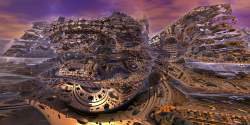News: Visit the official fractalforums.com Youtube Channel## The All New FractalForums is now in Public Beta Testing! Visit FractalForums.org and check it out!

baroquePrevious Image | Next Image
Description: this picture is only a fragment of a large picture, i failed several times to upload it here.
The complete picture should be available here(but as i found, again it is not completely available, but maybe it will give an impression of some spiralic features):
http://trajektorulm.tr.funpic.de/index.htm
made with an algorithm described at "more informations" on Youtube, video "artichoke"(the algo i wrote some years ago, it is implemented in QUASZ as gedatou(but only for power 2). the angles(measured from the northern pole and from meridian with geographic length zero) are multiplied with "power", as well as the distance of z1 is taken to the "power", the fragment of code goes as(the result is a trajectorial system just between the wonderful mandelbulb-algo and the squaring (or taking to higher powers) of quaternions:
a1 = x1 * x1 + y1 * y1 + z1 * z1;
//a2 = a1;//: REM  new distance if squaring only
a1 = sqrt(a1);
loa1=log(a1);
a2=exp(power*loa1);
yioi1 = y1 * y1 + z1 * z1;
yioi1 = sqrt(yioi1)         ;
//alpha1 = angle(x1, yioi1);
alpha1 = arg(x1+1i* yioi1);(would be in C++
alpha1=atan2(x1,yioi1))// if not, then atan2(yioi1,x1)
gamma1 = arg(y1+1i* z1);

alpha2 = power*alpha1;
gamma2 =power* gamma1                ;
x2 = cos(alpha2) * a2               ;
yioi2 = sin(alpha2) * a2        ;
y2 = (yioi2) *cos(gamma2);
z2 = (yioi2) * sin(gamma2) ;

Stats:
Total Favorities: 0 View Who Favorited
Filesize: 206.54kB
Height: 622 Width: 939
Keywords: mandelbulb mandelbrot set threedimensional fractals
Posted by: vectorJanuary 08, 2010, 05:50:51 PM

Rating:by 1 members.

 baroque carnivalRating: (None)Filesize: 337.71kBDate: July 10, 2009, 07:27:22 AMComments (0)By: Guest Baroque Brass TechRating:Filesize: 410.71kBDate: December 21, 2010, 06:16:37 PMComments (2)By: MarkJayBee